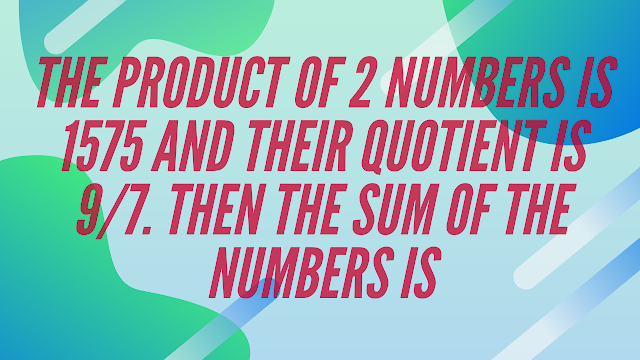# The product of 2 numbers is 1575 and their quotient is $\frac{9}{7}$. Then the sum of the numbers is

##Question:

The product of 2 numbers is 1575 and their quotient is $\frac{9}{7}$. Then the sum of the numbers is

a.   74

b.   78

c.   80

d.   90

Let the numbers be x and y.
$\therefore xy=1575$ and $\frac{x}{y}=\frac{9}{7}$
$\therefore \frac{xy}{(\frac{x}{y})}=\frac{1575}{(\frac{9}{7})}$
$\therefore y^2=1225$
$\therefore y=35$
Hence, $x=45$
So, sum of the numbers
$=x+y$
$=35+45$
$=80$